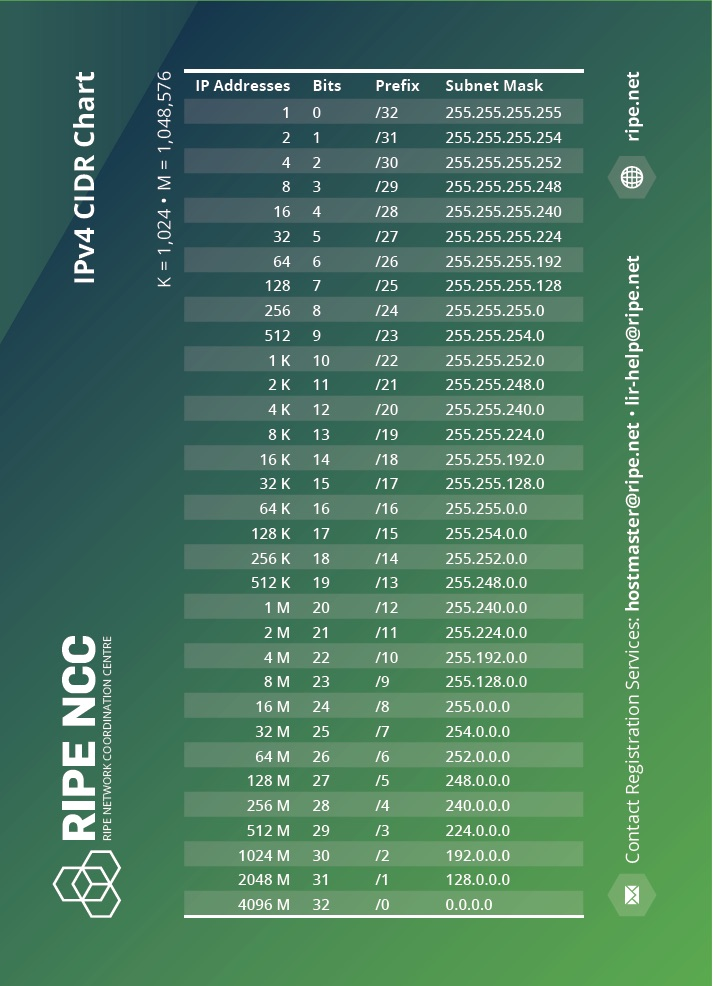# Understanding the IPv4 CIDR Chart

• September 22, 2023

## IPv4

The size of the prefix, in bits, is written after the oblique. This is called “slash notation”. There is a total of 32 bits in IPv4 address space. For example, if a network has the address “192.0.2.0/24”, the number “24” refers to how many bits are contained in the network. From this, the number of bits left for address space can be calculated. As all IPv4 networks have 32 bits, and each “section” of the address denoted by the decimal points contains eight bits, “192.0.2.0/24” leaves eight bits to contain host addresses. This is enough space for 256 host addresses. These host addresses are the IP addresses that are necessary to connect your machine to the Internet.

A network numbered “10.0.0.0/8” (which is one of those reserved for private use) is a network with eight bits of network prefix, denoted by “/8” after the oblique. The “8” denotes that there are 24 bits left over in the network to contain IPv4 host addresses: 16,777,216 addresses to be exact.

## Classless Inter-Domain Routing (CIDR) Chart

The Classless Inter-Domain Routing (CIDR) is commonly known as the CIDR chart and is used by those running networks and managing IP addresses. It enables them to see the number of IP addresses contained within each “slash notation” and the size of each “slash notation” in bits.